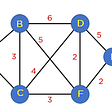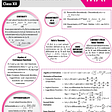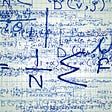# What are Conic Sections?

A curve, generated by intersecting a right circular cone with a plane is termed as ‘conic’. It has distinguished properties in Euclidean geometry. The vertex of the cone divides it into two nappes referred to as the upper nappe and the lower nappe. Conic sections are one of the important topics in Geometry. There are different types of conic sections in math that can be defined based on the angle formed between the plane and intersection of the right circular cone with it.

Now let’s take a look into important formulas and concepts from this chapter.

# Circle

A circle is the set of all points in a plane, which are at a fixed distance from a fixed point in the plane. The fixed point is called the center of the circle and the distance from center to any point on the circle is called the radius of the circle. The equation of a circle with radius r having center (h, k) is given by (x — h)2 + (y — k)2 = r2. The general equation of the circle is given by x2 + y2 + 2gx + 2fy + c = 0, where, g, f and c are constants.

• The center of the circle is (-g, -f).
• The radius of the circle is r = √g2+f2−c

The general equation of the circle passing through origin is x2 + y2 + 2gx + 2fy = 0.

The parametric equation of the circle x2 + y2 = r2 are given by x = r cos θ, y = r sin θ, where θ is the parameter and the parametric equation of the circle (x — h)2 + (y — k)2 = r2 are given by x = h + r cos θ, y = k + r sin θ.

# Parabola

A parabola is the set of points P whose distances from a fixed point F in the plane are equal to their distance from a fixed line l in the plane. The fixed point F is called focus and the fixed line l is the directrix of the parabola.

Important formulas for Parabola

# Ellipse

An ellipse is the set of all points in a plane such that the sum of whose distances from two fixed points is constant. Or to elaborate An ellipse is the set of all points in the plane whose distances from a fixed point in the plane bears a constant ratio, less than to their distance from a fixed point in the plane. The fixed point is called focus, the fixed line a directrix and the constant ratio(e) the eccentricity of the ellipse.

We have two standard forms of ellipse i.e.

Important formulas for Ellipse

# Hyperbola

A hyperbola is the locus of a point in a plane which moves in such a way that the ratio of its distance from a fixed point in the same plane to its distance from a fixed line is always constant which is always greater than unity. The fixed point is called the focus, the fixed line is called the directrix and the constant ratio, generally denoted bye, is known as the eccentricity of the hyperbola.

We have two standard forms of hyperbola i.e.

Important formulas for hyperbola

With this we’re at the end, now we know that not many students like this chapter. We get it! Few years back even we were there and we faced the fear of Conic Sections with an age old weapon, yes you got it right! “Practice”. In fact, not just conic sections, but any concept of Mathematics can be mastered by practicing them regularly. Now if you’re worried what if you encounter a doubt or a problem while practicing? Well that’s why we always have your back buddy! Just ask google chacha our address or simply click here and get the first 1v1 doubt session for free.

So don’t wait, go and practice questions and if you face a doubt, you know where to find us.

--

--

--

A doubt-solving platform for students, by students! Check out our website for more- doubtconnect.in

Love podcasts or audiobooks? Learn on the go with our new app.

## Kruskal Algorithm## Why you should learn mathematics?## Tips & Tricks for Continuity and Differentiability, Class 12th that’ll help you ace!## How to Read Without Distractions## Probability; Heads or Tails Class 12th## It’s not about mathematics anymore## How to Pick Winning Lottery Numbers Consistently## DoubtConnect

A doubt-solving platform for students, by students! Check out our website for more- doubtconnect.in

## Blink for The First Time## How to binning explorer in MatLab?## Automata Theory- Limitations and its Applications